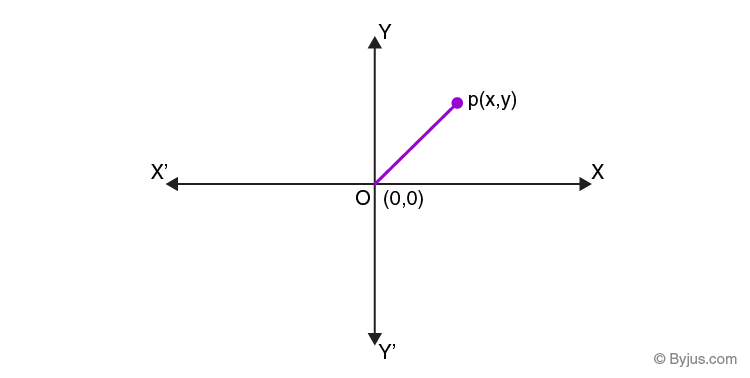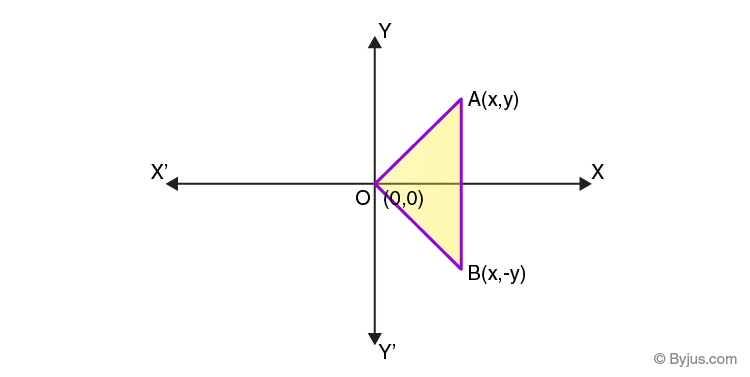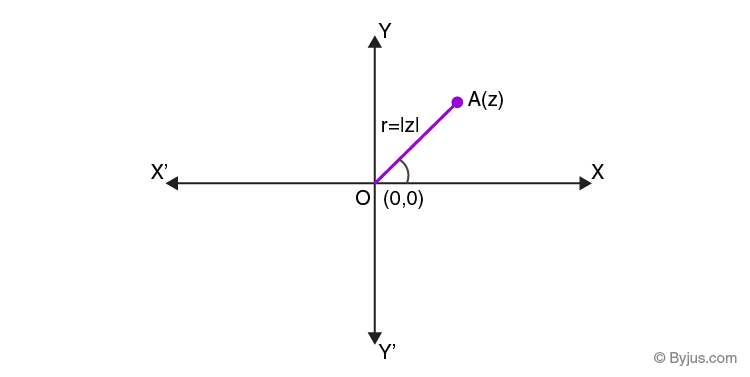Checkout JEE MAINS 2022 Question Paper Analysis : Checkout JEE MAINS 2022 Question Paper Analysis :

# Argand Plane & Polar Representation Of Complex Number

## Argand Plane

We all know that the pair of numbers (x, y) can be represented on the XY-plane, where x is called abscissa and y is called the ordinate. Similarly, we can represent complex numbers also on a plane called Argand plane or complex plane. Similar to the X-axis and Y-axis in two-dimensional geometry, there are two axes in Argand plane.

• The axis which is horizontal is called the real axis
• The axis which is vertical is called the imaginary axis

The complex number x+iy which corresponds to the ordered pair(x, y)is represented geometrically as the unique point (x, y) in the XY-plane.For example,

The complex number, 2+3i corresponds to the ordered pair (2, 3) geometrically.

Similarly, -3+2i corresponds to the ordered pair (-3, 2).

• Complex numbers in the form 0+ai, where “a” is any real number will lie on the imaginary axis.
• Complex numbers in the form a+0i, where “a” is any real number will lie on the real axis.

It is obvious that the modulus of complex number x+iy,

$$\begin{array}{l} \sqrt{x^2+y^2} \end{array}$$
is the distance between the origin (0, 0) and the point (x, y).

• The conjugate of z = x+iy is z = x-iy which is represented as (x, -y) in the Argand plane. Point (x, -y) is the mirror image of the point (x, y) across the real axis in the Argand plane.Example: Find the distance between the complex number z = 3 – 4i and the origin in the Argand plane.

Distance between the origin and z= 3 – 4i is equal to the modulus of z.

|z|=

$$\begin{array}{l} \sqrt{3^2+(-4)^2 }\end{array}$$
=
$$\begin{array}{l} \sqrt{9+16} \end{array}$$
=
$$\begin{array}{l}\sqrt{25}\end{array}$$
=5 units

Polar representation of complex numbers:Let “A” represent the non-zero complex number x + iy. OA is the directed line segment of length r and makes an angle θ with the positive direction of X-axis.

Ordered pair (r, θ) is called as the polar coordinates of the point A, as the point “A” is uniquely determined by (r, θ). The origin is called the pole and the positive X-axis is called the initial line.

Then,

x = r cosθ

y = r sinθ

We can write z = x + iy as z = r cosθ + ir sinθ = r(cosθ + i sinθ), which is called the polar form of complex number.

• Here, r = |z| =
$$\begin{array}{l} \sqrt{x^2+y^2}\end{array}$$
is modulus of z and θ is known as the argument or amplitude of z denoted as arg z
• For any non-zero complex number z, there corresponds one value of θ, in the interval [0, 2π)
• In any other interval of length 2π, for example, consider the interval -π < θ ≤ π, then the value of θ is called the principal argument of z.

Example: Represent z =

$$\begin{array}{l} \sqrt{3} \end{array}$$
+ i in the polar form

$$\begin{array}{l} \sqrt{3} \end{array}$$
= r cosθ

1=r sinθ

r = |z| =

$$\begin{array}{l} \sqrt{3 + 1} \end{array}$$
= 2

sin θ =

$$\begin{array}{l} \frac 12 \end{array}$$

cos θ =

$$\begin{array}{l} \frac {\sqrt{3}}{2}\end{array}$$

Which gives,

θ =

$$\begin{array}{l} \frac {\pi}{6} \end{array}$$

Therefore, polar form of z is,

z =

$$\begin{array}{l} 2~(cos~\left(\frac {\pi}{6} \right)~+~i~sin~\left(\frac{\pi}{6}\right)) \end{array}$$

To know more about many Maths-related topics, log onto www.byjus.com or download BYJU’S – The Learning App to learn with ease.